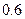Chapter D, Problem 23CTQ### Principles of Economics 2e

2nd Edition
Steven A. Greenlaw; David Shapiro
ISBN: 9781947172364

#### Solutions

Chapter
Section### Principles of Economics 2e

2nd Edition
Steven A. Greenlaw; David Shapiro
ISBN: 9781947172364
Textbook Problem

# Two counties are in a recession. Country A has an MPC ofand Country B has an MPC ofIn which country will government spending have the greatest impact?

To determine

The impact of government spending on two countries which have MPC of 0.8 and 0.6 respectively is to be determined.

Explanation

The value of spending multiplier can be computed by the following:

Spending Multiplier=11MPC

Thus, the spending multiplier for the country A having the MPC of 0.8 :

Spending Multiplier=11MPC=110.8=5

Now, the spending multiplier for the country B having the MPC of 0

### Still sussing out bartleby?

Check out a sample textbook solution.

See a sample solution

#### The Solution to Your Study Problems

Bartleby provides explanations to thousands of textbook problems written by our experts, many with advanced degrees!

Get Started

#### Find more solutions based on key concepts